# Bohr’s Model for Hydrogen Atom

Note: This model is applicable to H-atom or H-like species like He+, Li2+, Be3+.

## Postulates:

• An atom consists of a small, heavy, positively charged nucleus in the center and the electrons revolve around it in circular orbits.
• Electrons revolve only in those orbits which have a fixed value of energy. Hence, these orbits are called energy levels or stationary states.
They are numbered as 1,2,3,…… These numbers are known as Principal quantum Numbers.

### The energy of an electron is given by: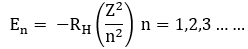Where RH is Rydberg’s constant and its value is 2.18 x 10-18 J.

Z = atomic number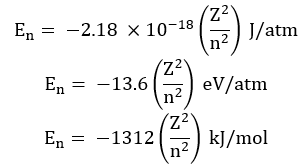Thus, energies of various levels are in the order:

K < L < M < N …… and so on.

The energy of the lowest state (n=1) is called ground state.

### Radii of the stationary states: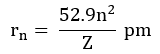For H-atom (Z=1), the radius of the first stationary state is called Bohr orbit (52.9 pm).

### Velocities of the electron in different orbit: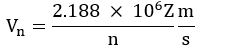• Since the electrons revolve only in those orbits which have fixed values of energy, hence electrons in an atom can have only certain definite values of energy and not any of their own. Thus, the energy of an electron is quantized.
• Like energy, the angular momentum of an electron in an atom can have certain definite values and not any value of their own.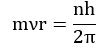Where n = 1,2,3…… and so on.

• An electron does not lose or gain energy when it is present in the same shell.
• When an electron gains energy, it gets excited to higher energy levels and when it de-excites, it loses energy in the form of electromagnetic radiations and comes to lower energy values.

## Limitations of Bohr’s model of the atom:

• Bohr’s model failed to account for the finer details of the hydrogen spectrum.
• Bohr’s model was also unable to explain the spectrum of atoms containing more than one electron.